# crossrun: An R Package for the Joint Distribution of Number of Crossings and Longest Run in Independent Bernoulli Observations

Centre for Child and Adolescent Mental Health, Eastern and Southern Norway, and Norwegian Centre of Violence and Traumatic Stress Studies
tore.wentzellarsen@gmail.com
Rigshospitalet, University of Copenhagen, Denmark
jacob@anhoej.net

## Introduction

The setting is defined by a number, n, of independent observations from a Bernoulli distribution with equal success probability. In statistical process control, our main intended application, this may be the useful observations in a run chart recording values above and below a pre-specified centre line (usually the median obtained from historical data) disregarding any observations equal to the centre line (Anhøj (2015)).

The focus of crossrun is the number of crossings, C, and the length of the longest run in the sequence, L. A run is a sequence of successes or failures, delimited by a different observation or the start or end of the entire sequence. A crossing is two adjacent different observations.

Figure 1 illustrates runs and crossings in a run chart with 24 observations. Observations above and below the median represent successes and failures respectively: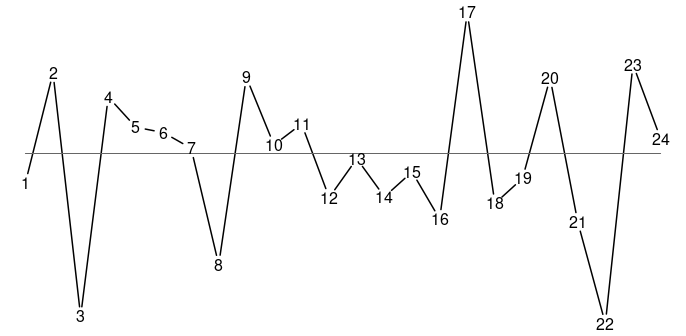Figure 1

The longest run consists of observations 12-16 below the median. Thus, the length of the longest run is L = 5 in this case. The number of crossings of the median is C = 11.

C and L are inversely related. All things being equal, when one goes up, the other goes down. crossrun computes the joint distribution of C and L.

C and L are integers. C may be any integer between 0, if all observations are equal, and n - 1 if all subsequent observations are different. L may be any integer between 1, if all subsequent observations are different, and n, if all observations are equal. Not all combinations of C and L are possible as shown in the following example for n = 14 and success probability = 0.5.

Table 1
L = 1 2 3 4 5 6 7 8 9 10 11 12 13 14
C = 0 0 0 0 0 0 0 0 0 0 0 0 0 0 1
1 0 0 0 0 0 0 1 2 2 2 2 2 2 0
2 0 0 0 0 3 12 18 15 12 9 6 3 0 0
3 0 0 0 10 58 78 60 40 24 12 4 0 0 0
4 0 0 5 130 230 175 100 50 20 5 0 0 0 0
5 0 0 90 456 405 210 90 30 6 0 0 0 0 0
6 0 1 392 735 392 147 42 7 0 0 0 0 0 0
7 0 28 756 644 224 56 8 0 0 0 0 0 0 0
8 0 126 756 324 72 9 0 0 0 0 0 0 0 0
9 0 210 405 90 10 0 0 0 0 0 0 0 0 0
10 0 165 110 11 0 0 0 0 0 0 0 0 0 0
11 0 66 12 0 0 0 0 0 0 0 0 0 0 0
12 0 13 0 0 0 0 0 0 0 0 0 0 0 0
13 1 0 0 0 0 0 0 0 0 0 0 0 0 0

As will be described and justified in more detail later, the table above does not give the probabilities themselves, but the probabilities multiplied by $$2^{n-1}$$, that is 8192 for n = 14. For instance P(C=6, L=5) = 392/8192 = 0.048. The highest joint probability is P (C=7, L=3) = P(C=8, L=3) = 756/8192 = 0.092. Thus, in a run chart with 14 observations not on the median, 7 or 8 crossings and longest run 3 constitute the most likely combination.

As seen in the table, the non-zero probabilities are confined to a sloped region that is rather narrow but sufficiently wide that the two variables together are more informative than each of them in isolation. These are general phenomena.

The procedure for computing the joint distribution of C and L is iterative, which means that the joint distribution for a sequence of length n cannot be computed before the joint distributions for all shorter sequences have been computed. The iterative procedure is described in detail in (Wentzel-Larsen and Anhøj (2019)). At the moment, the computations have been validated for n up to 100 and success probabilities 0.5 to 0.9 in steps of 0.1, and to some extent further up to n = 200 as detailed in (Wentzel-Larsen and Anhøj (2019)).

## The main function: crossrunbin

The function crossrunbin has two main arguments, nmax that is the maximum sequence length and prob that is the success probability. Other arguments include a multiplier mult described later that should normally be left at the default value 2, a precision parameter prec whose default value 120 has been checked to be sufficient up to n=100, and an additional argument printn that makes it possible to show progress information during the iterative calculations that are increasingly lengthy for increasing n. See ?crossrunbin for details.

The joint probabilities are stored in a list of 6 lists of which pt is sufficient for normal use. The others are mainly included for code checking. pt gives an n by n matrix for each sequence length n. For instance

crb40.6 <- crossrunbin(nmax = 40, prob = 0.6)$pt computes the joint probabilities for all sequence lengths $$n \leq 40$$ when the success probability is 0.6. For simplicity, the command above only returns the joint probabilities pt. When the computation is finished, the joint distribution for say n = 14 is crb40.6[] Actually the resulting joint distribution is not quite a matrix, it is a two-dimensional mpfr array (Fousse et, al, 2007, Mächler 2018). Two-dimensional mpfr arrays are almost the same as matrices, but with appreciably higher precision. Since the computation procedure is iterative, high precision during calculation is vital, but the resulting joint distributions may subsequently be transformed into ordinary matrices by the Rmpfr function asNumeric for easier presentation. To limit the package size, only the joint distributions for n = 14, 60, 100 and success probabilities 0.5 (the symmetric case) and 0.6 have been included in this package, as ordinary matrices. ## The “times” representation of the joint distributions As mentioned, the joint distributions are actually not computed as probabilities, but as probabilities times a factor whose default value is $$2^{n-1}$$. Optionally another factor $$m^{n-1}$$ could be used where $$m$$ is an argument (mult) to the function crossrunbin, but the default value should normally be used. This representation is shown in Table 1 above for n = 14 in the symmetric case p = 0.5. One may note that in Table 1 all probabilities are represented by integers in the “times” representation. This is a general phenomenon in the symmetric case, but not for success probabilities different from 0.5. In the symmetric case (Anhøj and Vingaard Olesen (2014)) the number, C, of crossings has a binomial distribution with number of observations n - 1 and probability 0.5, and the marginal probabilities are just the binomial coefficients divided by $$2^{n-1}$$. Indeed, the row sums in the times representation are the binomial coefficients in the symmetric case. This will be explicated later for n = 14. When the success probability is not 0.5, the joint distribution is no longer represented by integers even in the times representation. This is illustrated below for n = 14 and success probability 0.6. Table 2 L = 1 2 3 4 5 6 7 8 9 10 11 12 13 14 C = 0 0.0 0.0 0.0 0.0 0.0 0.0 0.0 0.0 0.0 0.0 0.0 0.0 0.0 6.4 1 0.0 0.0 0.0 0.0 0.0 0.0 0.8 1.6 2.0 2.8 4.0 5.8 8.6 0.0 2 0.0 0.0 0.0 0.0 3.4 15.7 29.3 27.2 25.2 22.5 18.4 11.5 0.0 0.0 3 0.0 0.0 0.0 7.9 53.0 85.7 76.5 60.2 44.0 27.4 11.6 0.0 0.0 0.0 4 0.0 0.0 4.5 131.1 263.2 227.9 148.0 86.6 41.5 12.7 0.0 0.0 0.0 0.0 5 0.0 0.0 73.4 413.2 414.6 243.7 121.7 48.5 11.9 0.0 0.0 0.0 0.0 0.0 6 0.0 0.8 354.5 727.9 430.8 182.5 60.7 12.0 0.0 0.0 0.0 0.0 0.0 0.0 7 0.0 21.8 637.1 591.8 228.5 65.5 11.0 0.0 0.0 0.0 0.0 0.0 0.0 0.0 8 0.0 104.6 666.1 308.9 76.3 10.9 0.0 0.0 0.0 0.0 0.0 0.0 0.0 0.0 9 0.0 166.7 337.7 81.2 10.1 0.0 0.0 0.0 0.0 0.0 0.0 0.0 0.0 0.0 10 0.0 134.5 93.6 10.1 0.0 0.0 0.0 0.0 0.0 0.0 0.0 0.0 0.0 0.0 11 0.0 51.5 9.8 0.0 0.0 0.0 0.0 0.0 0.0 0.0 0.0 0.0 0.0 0.0 12 0.0 10.2 0.0 0.0 0.0 0.0 0.0 0.0 0.0 0.0 0.0 0.0 0.0 0.0 13 0.8 0.0 0.0 0.0 0.0 0.0 0.0 0.0 0.0 0.0 0.0 0.0 0.0 0.0 In Table 2 the results are shown with one decimal. The cells different from 0 are the same as in the symmetric case, but the distribution centre has been shifted in the direction of longer runs and fewer crossings. The times representation may be advantageous for presentation because very small numbers are avoided. However, the main reason for using this representation is to enhance precision in the iterative computation procedure. ## The symmetric case: crossrunsymm In the symmetric case the joint probabilities are, as illustrated in Table 1, stored as integers in the times representation. A separate function crossrunsymm is available in this case. The arguments, except the success probability, are the same as in the more general function crossrunbin, but the inner workings are somewhat simpler. ## Generalisations In the case of variable success probability, a similar procedure is available and implemented in the function crossrunchange. In this procedure all arguments are as in crossrunbin, except that the success probability is replaced by a vector of length n with success probabilities for each of the n time points. Two other generalizations, to observations around the empirical median of the time series itself, and to autocorrelated time series, are the subject of the two following paragraphs. ## Empirical median For observations around the empirical median in the sequence itself the procedure in crossrunbin does not apply. A separate function crossrunem (where em represent empirical median) has been constructed for this case, and the code was first made available in (Wentzel-Larsen and Anhøj (2019)) before inclusion in crossrun. The setting may be assumed to originate in n independent and identical observations of a continuous variable, subsequently classified as above or below the median in the sequence itself. The useful observations, defined as the observations different from the empirical median (Anhøj and Vingaard Olesen (2014)), are always an even number, therefore the joint distribution of C and L is only needed for even n, say n=2m. Then, half the observations are by definition above the median and the others are below, and all $$2m \choose m$$ placements of the observations above the median are, by symmetry, equally probable. To compute the joint probabilities of C and L then amounts to determining the number of subsets of size m=n/2 for each combination of C and L. In analogy with the times representation it is the number of subsets that is computed and stored and not the probabilities. For these computations, an iterative procedure resembling the corresponding procedure in crossrunbin has been developed. For the procedure to work it has been necessary to compute the number of subsets of any size m, $$0 \leq m \leq n$$, for each combination of C and L, and also perform the computations for odd n. Since the result is only of interest for even n and for m=n/2, the numbers of interest thus amount to a tiny part of what actually has to be computed to make the procedure work. In addition, the number of subsets containing and not containing the first observation have to be computed separately for each combination of C and L. For m=n/2 these two numbers are equal by symmetry, but this is not so for other subset sizes m. The procedure is still appreciably less demanding in terms of storage and computer time than procedures based on explicit enumeration of all subsets. It is, however, much more demanding than the procedure implemented in crossrunbin due to the large number of subsets of different sizes m. For even n and m=n/2, the only case of actual interest, the total number of subsets, summing over all possible values of C and L, is $$2m \choose m$$, and by symmetry the total number of subsets including the first observation is the half of that, $${2m \choose m} / 2$$. Probabilities are then computed by dividing the numbers actually computed and stored by this number. The function crossrunem has arguments nmax for maximum sequence length, prec for precision in the Rmpfr computations, and printn for including progress information during the computations. The procedure has so far been possible to use only up to nmax=64 due to practical limitations in terms of computer time and storage. A function crossrunemcont has been made for extension of the results of an existing crossrunem computation, so that one does not need to start from scratch when attempting to extend the results of the computations to a somewhat higher value of n. As an illustration, the resulting distribution of C and L is shown below for n=14. Then there are 7 observations on both sides of the empirical median. The number of crossings C may still be as high as n-1=13, but there has to be at least one crossing. Also, the longest run cannot be larger than 7. Table 3 l=1 l=2 l=3 l=4 l=5 l=6 l=7 c=1 0 0 0 0 0 0 1 c=2 0 0 0 0 0 0 6 c=3 0 0 0 4 12 20 0 c=4 0 0 0 24 36 30 0 c=5 0 0 36 108 81 0 0 c=6 0 0 96 144 60 0 0 c=7 0 16 240 144 0 0 0 c=8 0 40 200 60 0 0 0 c=9 0 100 125 0 0 0 0 c=10 0 60 30 0 0 0 0 c=11 0 36 0 0 0 0 0 c=12 0 6 0 0 0 0 0 c=13 1 0 0 0 0 0 0 This table represents the partitioning by C and L of the total number of subsets of size 7 of 14 observations, including the first observation. This total number is $${14 \choose 7} / 2 = 1716$$. The corresponding probabilities of each combination of C and L are obtained by dividing by 1716. For example, $$P (C=5, L=4) = 108/1716 = 0.063$$. The combination with the highest probability is C=7, L=3 with probability 240/1716 = 0.140. The corresponding joint distribution of C and L with a predetermined midline is shown in Table 1 above in the symmetric case. The two distributions have some similarity although there are fewer possible combinations with the empirical median. The following diagrams compare the marginal distributions of C and L for n=14 and for n=60. At least for the marginal distributions the differences seem to be lower for n=60 than for n=14.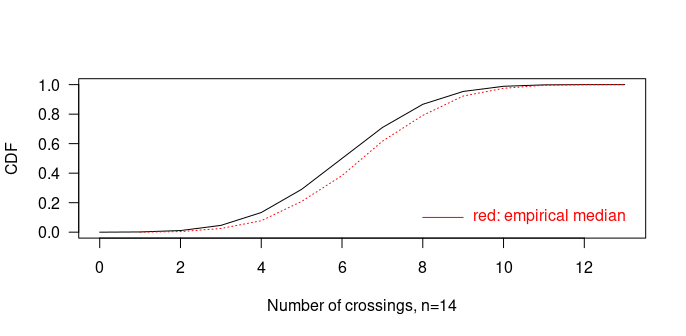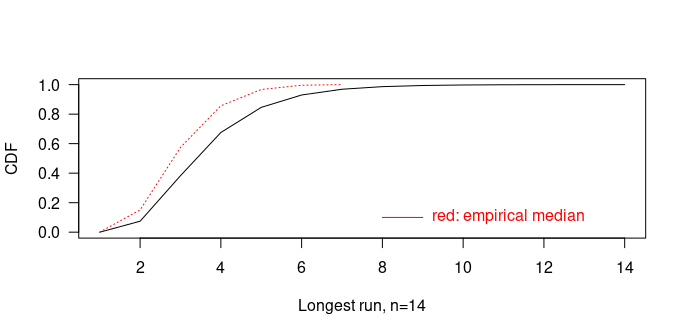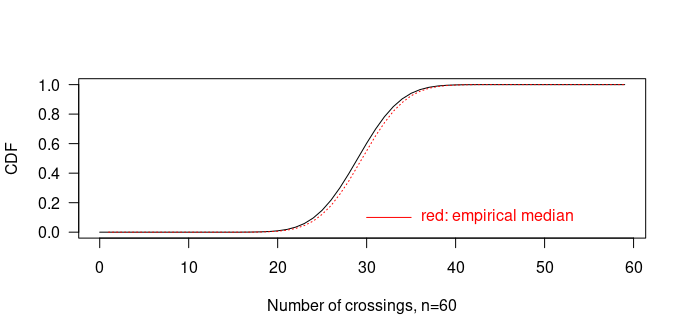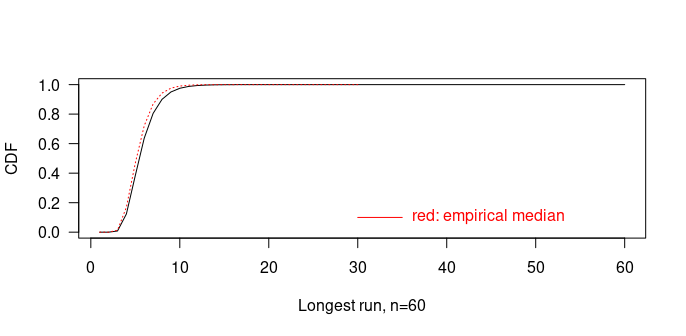## Autocorrelated observations auto ## Conclusions The crossrun package is, to our knowledge the first software package that allows for the computation of joint probabilities of longest run and number of crossings in time series data. This is an important step forward, as previous work on the subject have only dealt with these parameters as independent entities. This work may form the basis of better tests for non-random variation in time series data than are currently available. ## Appendix: Checking procedures Procedures for checking the joint distributions are available in crossrun. First, the function exactbin computes the joint distribution for $$n \leq 6$$ independently of the iterative procedure, by formulas based on “brute force” enumeration that is practically feasible for these short sequences. An example of use of exactbin for checking of results of the exact procedure is as follows, for n=5 and success probability 0.6 (multiplied by $$2^4=16$$ to conform with the times representation): library(crossrun) library(Rmpfr) exact1 <- asNumeric((2^4) * exactbin(n = 5, p = 0.6)) iter1 <- asNumeric(crossrunbin(nmax = 5, prob = 0.6)$pt[])
compare1 <- cbind(exact1,iter1)

compare1
#>        1      2      3      4     5      1      2      3      4     5
#> 0 0.0000 0.0000 0.0000 0.0000 1.408 0.0000 0.0000 0.0000 0.0000 1.408
#> 1 0.0000 0.0000 1.8432 2.1504 0.000 0.0000 0.0000 1.8432 2.1504 0.000
#> 2 0.0000 2.9184 3.0720 0.0000 0.000 0.0000 2.9184 3.0720 0.0000 0.000
#> 3 0.0000 3.6864 0.0000 0.0000 0.000 0.0000 3.6864 0.0000 0.0000 0.000
#> 4 0.9216 0.0000 0.0000 0.0000 0.000 0.9216 0.0000 0.0000 0.0000 0.000

Here the 5 leftmost columns come from exact calculations while the 5 rightmost columns come from the iterative procedure. The maximum absolute difference is computed as 0 in this case. Generally some tiny differences may occur.

The iterative computations may also be checked for appreciably higher n. As commented above the row sums in the symmetric case are just the binomial coefficients. The following code checks this fact for n=14.

library(crossrun)
library(Rmpfr)

bincoeff13           <- Rmpfr::chooseMpfr.all(13) # binomial coefficients, n - 1 = 13
bincoeff13iter       <- cumsumm(j14s)[-1, 14]     # row sums, n - 1 = 13
compare13            <- rbind(asNumeric(bincoeff13), bincoeff13iter)
row.names(compare13) <- c("exact","iter")
compare13
#>        1  2   3   4    5    6    7    8   9  10 11 12 13
#> exact 13 78 286 715 1287 1716 1716 1287 715 286 78 13  1
#> iter  13 78 286 715 1287 1716 1716 1287 715 286 78 13  1
max(abs(bincoeff13 - bincoeff13iter))
#> 1 'mpfr' number of precision  53   bits
#>  0

This check has been repeated for $$n \leq 100$$ with full mpfr precision without finding any discrepancies.

The results of the iterative procedure may also be checked by results of simulations. The crossrun function simclbin performs simulations for the number of crossings and the longest run for chosen values of the success probability. Again, computations for substantially longer sequences (n appreciably higher than 14) should use full mpfr precision for the joint distribution. The following code shows the procedure for n = 14 and 10000 simulations and compares the mean of $$C \cdot L$$ in the simulations with the corresponding mean from the joint distribution p14.6 with success probability 0.6:

library(crossrun)
set.seed(83938487)
sim14 <- simclbin(nser = 14, nsim = 10000)
(matrix(0:13, nrow = 1) %*% p14.6%*% matrix(1:14, ncol = 1)) / 2^13
#>          [,1]
#> [1,] 25.47043
mean(sim14$nc0.6 * sim14$lr0.6)
#>  25.4656

Here, $$C \cdot L$$ is just used as an example of a fairly demanding function of the number C of crossing and the longest run L. Again, computations for substantially longer sequences (n appreciably higher than 14) should use full mpfr precision for the joint distribution. Simpler statistics include means and standard deviations of C and L separately. The following shows a graphical comparison of the cumulative distribution functions of C and L based on the joint distribution and the simulations.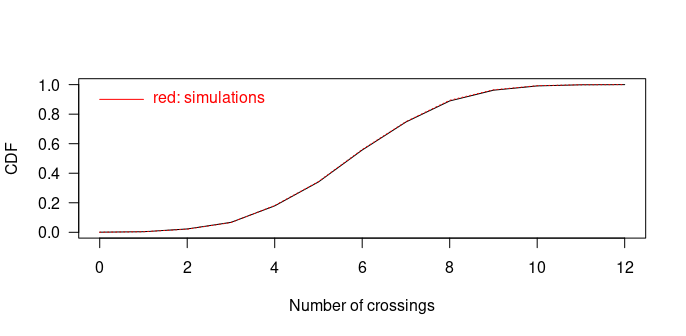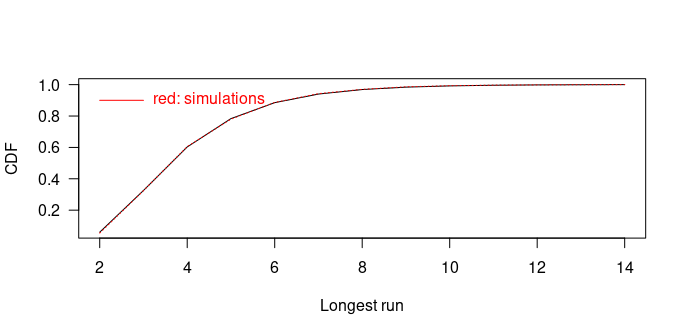The joint distribution of C and L may also be checked by simulations when the empirical median is used. A function simclem repeatedly generates n=2m independent observations from the standard normal, computes their median and computes C and L from the resulting sequence. The result is an empirical distribition of C and L among the simulations. The result is illustrated for n=14 and the number of simulations equal to $$100 \cdot 1716$$ to ease comparison with the exact distribution shown in Table 3 above. In one run the empirical distribution, divided by 100 is as follows

Table 4
L=1 L=2 L=3 L=4 L=5 L=6 L=7
C=1 0.0 0.0 0.0 0.0 0.0 0.0 1.1
C=2 0.0 0.0 0.0 0.0 0.0 0.0 6.0
C=3 0.0 0.0 0.0 4.3 11.7 19.6 0.0
C=4 0.0 0.0 0.0 24.1 36.3 30.2 0.0
C=5 0.0 0.0 37.2 108.3 81.3 0.0 0.0
C=6 0.0 0.0 96.0 142.9 59.6 0.0 0.0
C=7 0.0 16.0 238.5 144.5 0.0 0.0 0.0
C=8 0.0 39.5 200.1 59.7 0.0 0.0 0.0
C=9 0.0 99.9 124.4 0.0 0.0 0.0 0.0
C=10 0.0 61.1 30.9 0.0 0.0 0.0 0.0
C=11 0.0 36.0 0.0 0.0 0.0 0.0 0.0
C=12 0.0 5.9 0.0 0.0 0.0 0.0 0.0
C=13 0.9 0.0 0.0 0.0 0.0 0.0 0.0

Generally this is in good agreement with Table 3. For n=60 a figure is more informative, based on the default 10000 simulations and only checking the marginal distributions.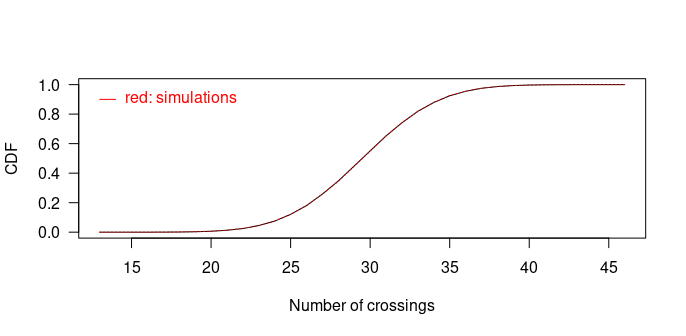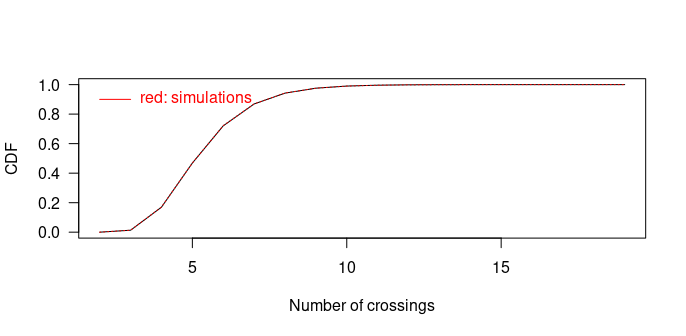There is good agreement with the simulations.